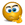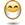# Gilt Curve

## Excel Help for Gilt Curve in Valuing Investments and Businesses

### Gilt Curve

Rate this:
(3/5 from 1 vote)HiLooking to find some cunning VBA code to build a Gilt curve using some sort of cubic spline. Ben Posted by Ben on 09 Feb 2007
 Replies - Displaying 1 to 3 of 3 Order Replies By: Most Recent | Chronological | Highest RatedRate this:
You can create a user defined function to calculate interpolated values of the gilt curve using a cubic spline.  The following spreadsheet shows the calculation of a cubic spline interpolated value given known values for fixed periods.

 A B C D E 1 Period: Rate: X Period: Spline Value: 2 1 5.2 1.2 =spline(\$A\$2:\$A\$4,\$B\$2:\$B\$4,D2) 3 2 4.3 4 3 6.5

The value of the calculated spline value for the interpolated period will be 4.87.  Columns D and E can be copied down to create the entire curve for the X periods required in column D.

Of course the spline funtion first needs to added to a VBA project.  The spline function is as follows.

VBA Code:
 Function spline(periodcol As Range, ratecol As Range, x As Range)Dim period_count As IntegerDim rate_count As Integerperiod_count = periodcol.Rows.Countrate_count = ratecol.Rows.CountIf period_count <> rate_count Then    spline = "Error: Range count dos not match"    GoTo endnowEnd If ReDim xin(period_count) As SingleReDim yin(period_count) As SingleDim c As IntegerFor c = 1 To period_countxin(c) = periodcol(c)yin(c) = ratecol(c)Next cDim n As IntegerDim i, k As IntegerDim p, qn, sig, un As SingleReDim u(period_count - 1) As SingleReDim yt(period_count) As Singlen = period_countyt(1) = 0u(1) = 0For i = 2 To n - 1    sig = (xin(i) - xin(i - 1)) / (xin(i + 1) - xin(i - 1))    p = sig * yt(i - 1) + 2    yt(i) = (sig - 1) / p    u(i) = (yin(i + 1) - yin(i)) / (xin(i + 1) - xin(i)) - (yin(i) - yin(i - 1)) / (xin(i) - xin(i - 1))    u(i) = (6 * u(i) / (xin(i + 1) - xin(i - 1)) - sig * u(i - 1)) / p        Next i    qn = 0un = 0yt(n) = (un - qn * u(n - 1)) / (qn * yt(n - 1) + 1)For k = n - 1 To 1 Step -1    yt(k) = yt(k) * yt(k + 1) + u(k)Next kDim klo, khi As IntegerDim h, b, a As Singleklo = 1khi = nDok = khi - kloIf xin(k) > x Thenkhi = kElseklo = kEnd Ifk = khi - kloLoop While k > 1h = xin(khi) - xin(klo)a = (xin(khi) - x) / hb = (x - xin(klo)) / hy = a * yin(klo) + b * yin(khi) + ((a ^ 3 - a) * yt(klo) + (b ^ 3 - b) * yt(khi)) * (h ^ 2) / 6spline = yendnow:End FunctionRate this: (3/5 from 1 vote) hi I guess that this code assumes "zero" value for the second derivatives of the first and last points of the data which are needed as the boundary conditions. Am I right? thanks Posted by hfml on 30 Apr 2017Rate this: (3.5/5 from 2 votes) The VBA function does assume zero values for the first and last points of the boundaries . Excel Business Forums Administrator Posted by Excel Helper on 30 Apr 2017